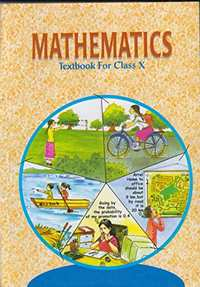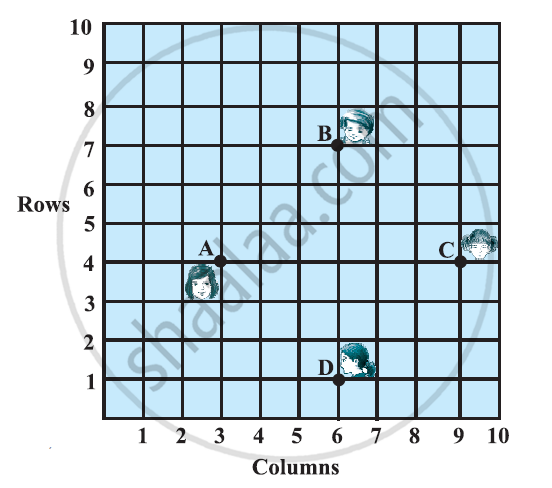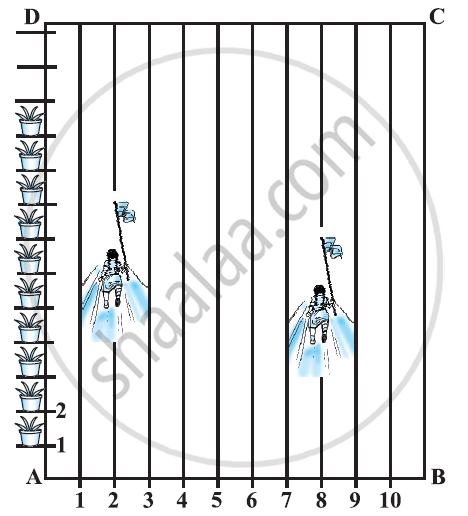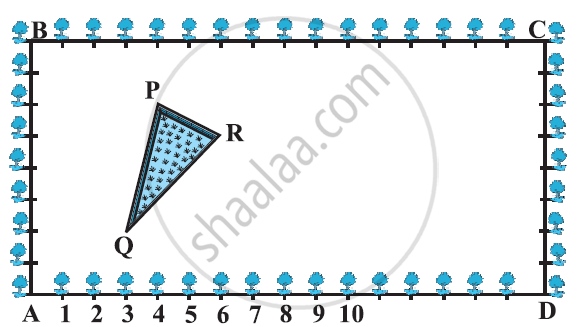Share

NCERT solutions for Class 10 Mathematics chapter 7 - Coordinate Geometry

Mathematics Textbook for Class 10

NCERT Mathematics Class 10Chapter 7: Coordinate Geometry

Ex. 7.10Ex. 7.20Ex. 7.30Ex. 7.40

Chapter 7: Coordinate Geometry Exercise 7.10 solutions [Pages 161 - 162]

Ex. 7.10 | Q 1.1 | Page 161

Find the distance between the following pair of point (2, 3), (4, 1)

Ex. 7.10 | Q 1.2 | Page 161

Find the distance between the following pairs of points: (−5, 7), (−1, 3)

Ex. 7.10 | Q 1.3 | Page 161

Find the distance between the following pair of point:(ab), (− a, − b)

Ex. 7.10 | Q 2 | Page 161

Find the distance between the points (0, 0) and (36, 15). Can you now find the distance between the two towns A and B discussed in Section 7.2.

Ex. 7.10 | Q 3 | Page 161

Determine if the points (1, 5), (2, 3) and (− 2, − 11) are collinear.

Ex. 7.10 | Q 4 | Page 161

Check whether (5, - 2), (6, 4) and (7, - 2) are the vertices of an isosceles triangle.

Ex. 7.10 | Q 5 | Page 161

In a classroom, 4 friends are seated at the points A, B, C and D as shown in the following figure. Champa and Chameli walk into the class and after observing for a few minutes Champa asks Chameli, “Don’t you think ABCD is a square?” Chameli disagrees.

Using distance formula, find which of them is correct.Ex. 7.10 | Q 6.1 | Page 161

Name the type of quadrilateral formed, if any, by the given points, and give reasons for your answer: (- 1, - 2), (1, 0), (- 1, 2), (- 3, 0)

Ex. 7.10 | Q 6.2 | Page 161

Name the type of quadrilateral formed, if any, by the following point, and give reasons for your answer: (− 3, 5), (3, 1), (0, 3), (− 1, − 4)

Ex. 7.10 | Q 6.3 | Page 161

Name the type of quadrilateral formed, if any, by the following point, and give reasons for your answer (4, 5), (7, 6), (4, 3), (1, 2)

Ex. 7.10 | Q 7 | Page 161

Find the point on the x-axis which is equidistant from (2, - 5) and (- 2, 9).

Ex. 7.10 | Q 8 | Page 161

Find the values of y for which the distance between the points P (2, - 3) and Q (10, y) is 10 units

Ex. 7.10 | Q 9 | Page 162

If Q (0, 1) is equidistant from P (5, − 3) and R (x, 6), find the values of x. Also find the distance QR and PR.

Ex. 7.10 | Q 10 | Page 162

Find a relation between x and y such that the point (xy) is equidistant from the point (3, 6) and (− 3, 4).

Chapter 7: Coordinate Geometry Exercise 7.20 solutions [Page 167]

Ex. 7.20 | Q 1 | Page 167

Find the coordinates of the point which divides the join of (–1, 7) and (4, –3) in the ratio 2 : 3.

Ex. 7.20 | Q 2 | Page 167

Find the coordinates of the points of trisection of the line segment joining (4, -1) and (-2, -3).

Ex. 7.20 | Q 3 | Page 167

To conduct Sports Day activities, in your rectangular shaped school ground ABCD, lines have been drawn with chalk powder at a distance of 1 m each. 100 flower pots have been placed at a distance of 1 m from each other along AD, as shown in the following figure. Niharika runs 1/4 th the distance AD on the 2nd line and posts a green flag. Preet runs 1/5

th the distance AD on the eighth line and posts a red flag. What is the distance between both the flags? If Rashmi has to post a blue flag exactly halfway between the line segment joining the two flags, where should she post her flag?Ex. 7.20 | Q 4 | Page 167

Find the ratio in which the line segment joining the points (-3, 10) and (6, - 8) is divided by (-1, 6).

Ex. 7.20 | Q 5 | Page 167

Find the ratio in which the line segment joining A (1, − 5) and B (− 4, 5) is divided by the x-axis. Also find the coordinates of the point of division.

Ex. 7.20 | Q 6 | Page 167

If (1, 2), (4, y), (x, 6) and (3, 5) are the vertices of a parallelogram taken in order, find x and y.

Ex. 7.20 | Q 7 | Page 167

Find the coordinates of a point A, where AB is the diameter of circle whose centre is (2, − 3) and B is (1, 4)

Ex. 7.20 | Q 8 | Page 167

If A and B are (− 2, − 2) and (2, − 4), respectively, find the coordinates of P such that AP = 3/7 AB and P lies on the line segment AB.

Ex. 7.20 | Q 9 | Page 167

Find the coordinates of the points which divide the line segment joining A (- 2, 2) and B (2, 8) into four equal parts.

Ex. 7.20 | Q 10 | Page 167

Find the area of a rhombus if its vertices are (3, 0), (4, 5), (− 1, 4) and (− 2, −1) taken in order. [Hint: Area of a rhombus = 1/2 (product of its diagonals)]

Chapter 7: Coordinate Geometry Exercise 7.30 solutions [Page 170]

Ex. 7.30 | Q 1.1 | Page 170

Find the area of the triangle whose vertices are: (2, 3), (-1, 0), (2, -4)

Ex. 7.30 | Q 1.2 | Page 170

Find the area of the triangle whose vertices are: (–5, –1), (3, –5), (5, 2)

Ex. 7.30 | Q 2.1 | Page 170

In each of the following find the value of 'k', for which the points are collinear.

(7, -2), (5, 1), (3, -k

Ex. 7.30 | Q 2.2 | Page 170

In each of the following find the value of 'k', for which the points are collinear.

(8, 1), (k, -4), (2, -5)

Ex. 7.30 | Q 3 | Page 170

Find the area of the triangle formed by joining the mid-points of the sides of the triangle whose vertices are (0, -1), (2, 1) and (0, 3). Find the ratio of this area to the area of the given triangle

Ex. 7.30 | Q 4 | Page 170

Find the area of the quadrilateral whose vertices, taken in order, are (-4, -2), (-3, -5), (3, -2) and (2, 3).

Ex. 7.30 | Q 5 | Page 170

median of a triangle divides it into two triangles of equal areas. Verify this result for ΔABC whose vertices are A (4, - 6), B (3, - 2) and C (5, 2).

Chapter 7: Coordinate Geometry Exercise 7.40 solutions [Pages 171 - 172]

Ex. 7.40 | Q 1 | Page 171

Determine the ratio in which the line 2x + y – 4 = 0 divides the line segment joining the points A(2, – 2) and B(3, 7).

Ex. 7.40 | Q 2 | Page 171

Find a relation between x and y if the points (x, y), (1, 2) and (7, 0) are collinear.

Ex. 7.40 | Q 3 | Page 171

Find the centre of a circle passing through the points (6, − 6), (3, − 7) and (3, 3).

Ex. 7.40 | Q 4 | Page 171

The two opposite vertices of a square are (− 1, 2) and (3, 2). Find the coordinates of the other two vertices.

Ex. 7.40 | Q 5 | Page 171

The class X students of a secondary school in Krishinagar have been allotted a rectangular plot of land for their gardening activity. Saplings of Gulmohar are planted on the boundary at a distance of 1 m from each other. There is a triangular grassy lawn in the plot as shown in the following figure. The students are to sow seeds of flowering plants on the remaining area of the plot.(i) Taking A as origin, find the coordinates of the vertices of the triangle.

(ii) What will be the coordinates of the vertices of Δ PQR if C is the origin?

Also calculate the areas of the triangles in these cases. What do you observe?

Ex. 7.40 | Q 6 | Page 171

The vertices of a ΔABC are A (4, 6), B (1, 5) and C (7, 2). A line is drawn to intersect sides AB and AC at D and E respectively, such that (AD)/(AB) = (AE)/(AC) = 1/4Calculate the area of the ΔADE and compare it with the area of ΔABC. (Recall Converse of basic proportionality theorem and Theorem 6.6 related to ratio of areas of two similar triangles)

Ex. 7.40 | Q 7 | Page 171

Let A (4, 2), B (6, 5) and C (1, 4) be the vertices of ΔABC.

(i) The median from A meets BC at D. Find the coordinates of point D.

(ii) Find the coordinates of the point P on AD such that AP: PD = 2:1

(iii) Find the coordinates of point Q and R on medians BE and CF respectively such that BQ: QE = 2:1 and CR: RF = 2:1.

(iv) What do you observe?

(v) If A(x1y1), B(x2y2), and C(x3y3) are the vertices of ΔABC, find the coordinates of the centroid of the triangle.

Ex. 7.40 | Q 8 | Page 172

ABCD is a rectangle formed by the points A(-1, -1), B(-1, 4), C(5, 4) and D(5, -1). P, Q, R and S are the midpoints of AB, BC, CD and DA respectively. Is the quadrilateral PQRS a square? a rectangle? or a rhombus? Justify your answer.

Chapter 7: Coordinate Geometry

Ex. 7.10Ex. 7.20Ex. 7.30Ex. 7.40

NCERT Mathematics Class 10NCERT solutions for Class 10 Mathematics chapter 7 - Coordinate Geometry

NCERT solutions for Class 10 Maths chapter 7 (Coordinate Geometry) include all questions with solution and detail explanation. This will clear students doubts about any question and improve application skills while preparing for board exams. The detailed, step-by-step solutions will help you understand the concepts better and clear your confusions, if any. Shaalaa.com has the CBSE Mathematics Textbook for Class 10 solutions in a manner that help students grasp basic concepts better and faster.

Further, we at Shaalaa.com are providing such solutions so that students can prepare for written exams. NCERT textbook solutions can be a core help for self-study and acts as a perfect self-help guidance for students.

Concepts covered in Class 10 Mathematics chapter 7 Coordinate Geometry are Section Formula, Area of a Triangle, Graphs of Linear Equations, Coordinate Geometry Examples and Solutions, Distance Formula, Concepts of Coordinate Geometry.

Using NCERT Class 10 solutions Coordinate Geometry exercise by students are an easy way to prepare for the exams, as they involve solutions arranged chapter-wise also page wise. The questions involved in NCERT Solutions are important questions that can be asked in the final exam. Maximum students of CBSE Class 10 prefer NCERT Textbook Solutions to score more in exam.

Get the free view of chapter 7 Coordinate Geometry Class 10 extra questions for Maths and can use Shaalaa.com to keep it handy for your exam preparation

S